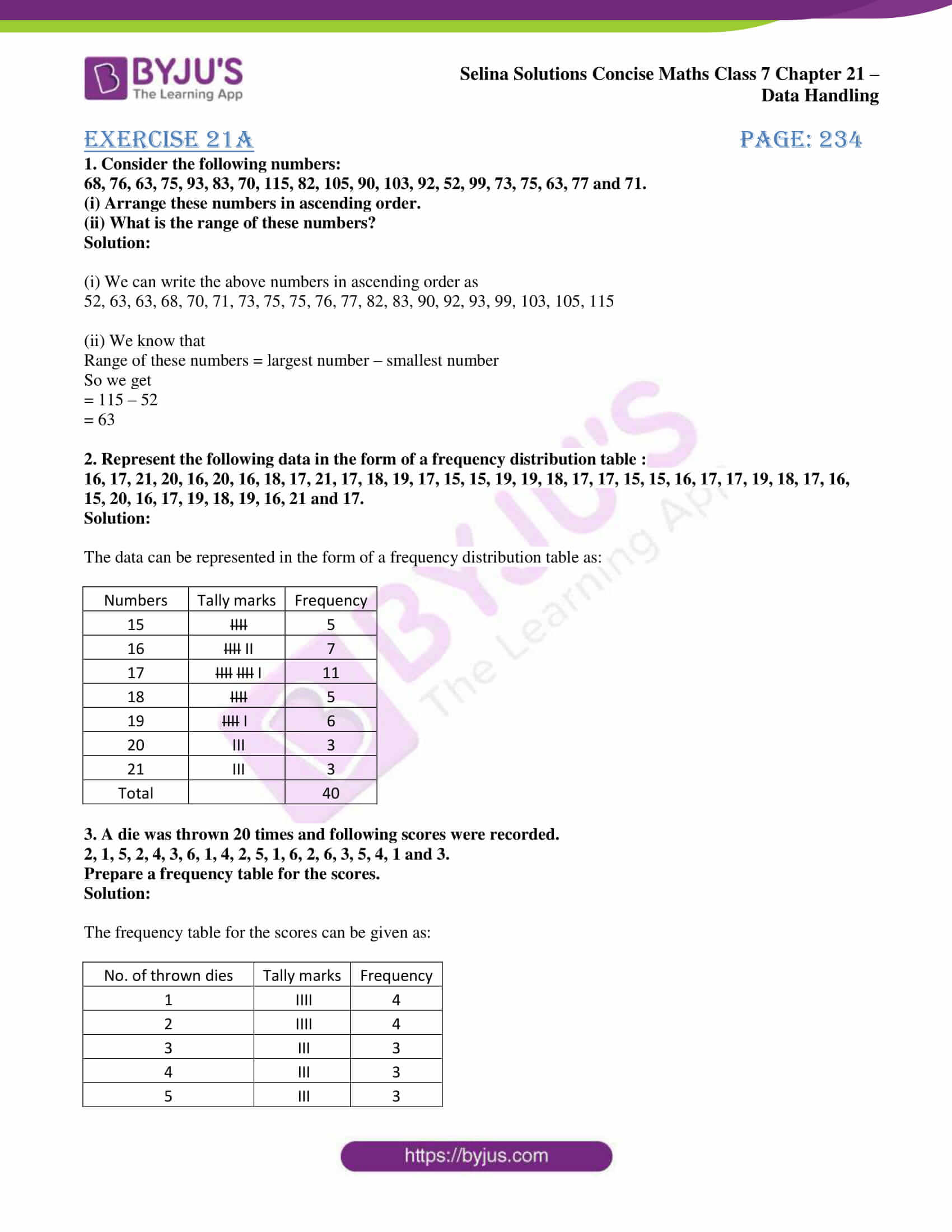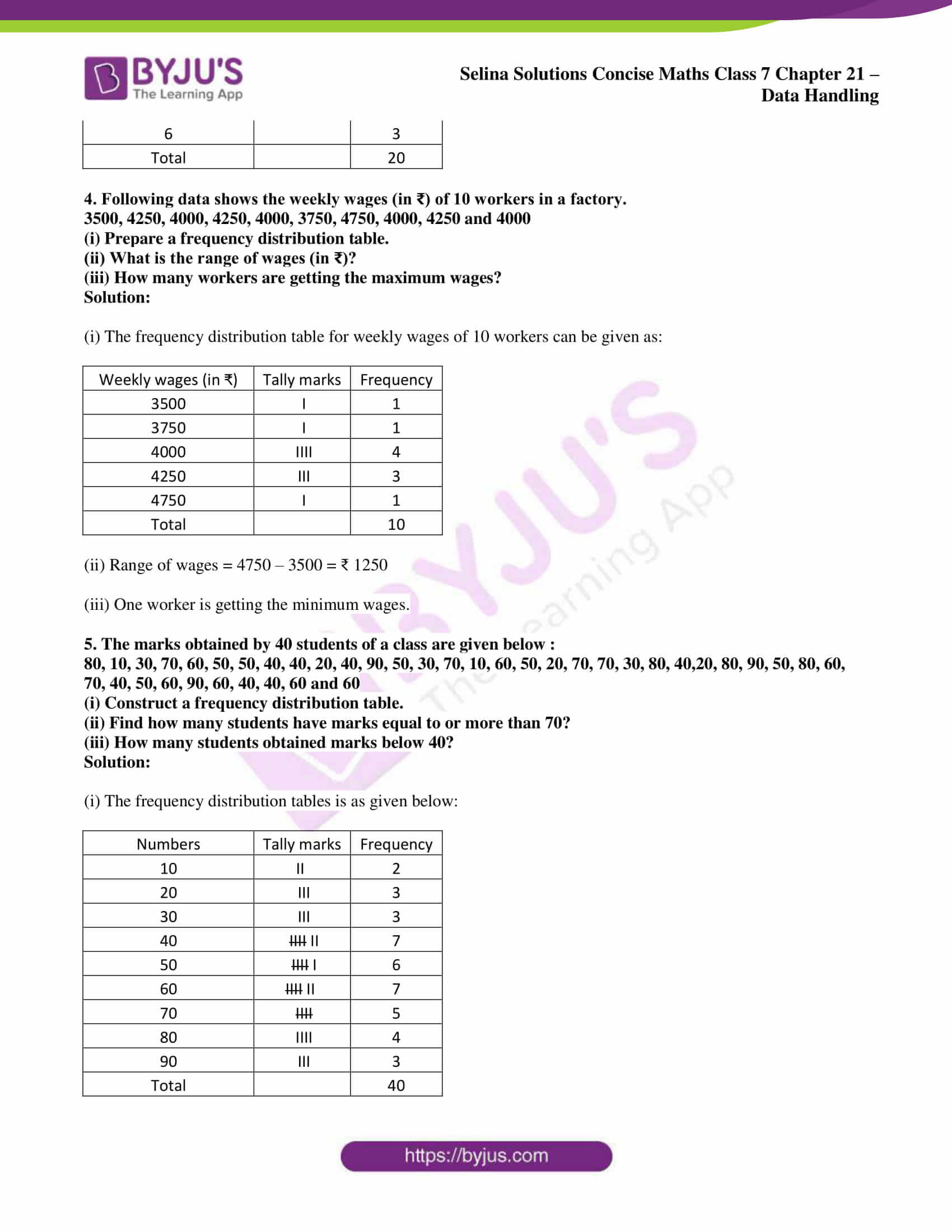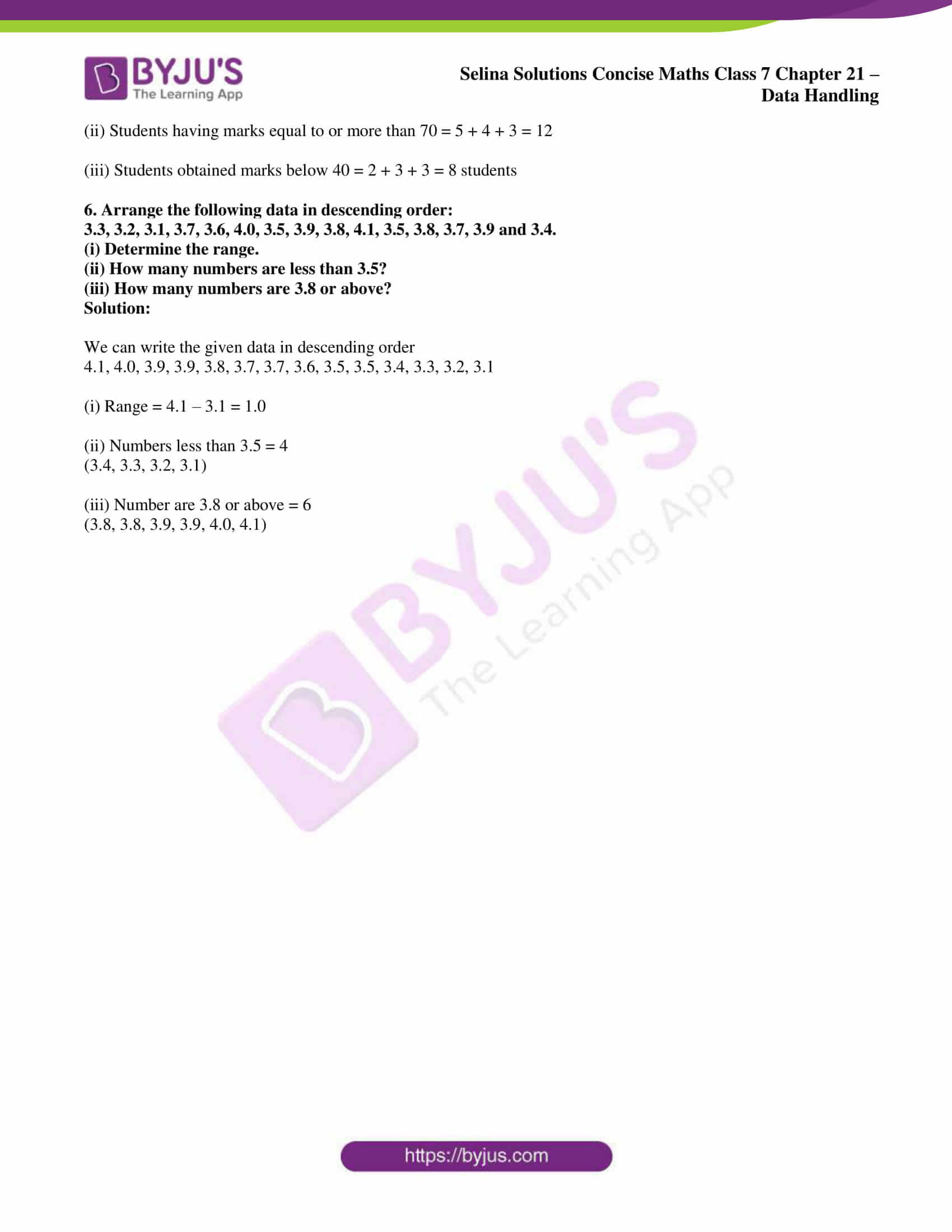# Selina Solutions Concise Maths Class 7 Chapter 21: Data Handling Exercise 21A

Selina Solutions Concise Maths Class 7 Chapter 21 Data Handling Exercise 21A are created to nurture the minds of students about the concepts covered in this chapter. Data Handling is one of the main topics, which would also be continued even in further classes. The method of handling the given set of data is discussed comprehensively in Exercise 21A. Students are advised to solve the exercise wise problems using the solutions PDF to score well in the annual exam. Selina Solutions Concise Maths Class 7 Chapter 21 Data Handling Exercise 21A, free PDF links are provided below with a download option.

## Selina Solutions Concise Maths Class 7 Chapter 21: Data Handling Exercise 21A Download PDF### Access Selina Solutions Concise Maths Class 7 Chapter 21: Data Handling Exercise 21A

Exercise 21A page: 234

1. Consider the following numbers:
68, 76, 63, 75, 93, 83, 70, 115, 82, 105, 90, 103, 92, 52, 99, 73, 75, 63, 77 and 71.
(i) Arrange these numbers in ascending order.
(ii) What is the range of these numbers?

Solution:

(i) We can write the above numbers in ascending order as

52, 63, 63, 68, 70, 71, 73, 75, 75, 76, 77, 82, 83, 90, 92, 93, 99, 103, 105, 115

(ii) We know that

Range of these numbers = largest number – smallest number

So we get

= 115 – 52

= 63

2. Represent the following data in the form of a frequency distribution table :

16, 17, 21, 20, 16, 20, 16, 18, 17, 21, 17, 18, 19, 17, 15, 15, 19, 19, 18, 17, 17, 15, 15, 16, 17, 17, 19, 18, 17, 16, 15, 20, 16, 17, 19, 18, 19, 16, 21 and 17.

Solution:

The data can be represented in the form of a frequency distribution table as:

 Numbers Tally marks Frequency 15 IIII 5 16 IIII II 7 17 IIII IIII I 11 18 IIII 5 19 IIII I 6 20 III 3 21 III 3 Total 40

3. A die was thrown 20 times and following scores were recorded.

2, 1, 5, 2, 4, 3, 6, 1, 4, 2, 5, 1, 6, 2, 6, 3, 5, 4, 1 and 3.

Prepare a frequency table for the scores.

Solution:

The frequency table for the scores can be given as:

 No. of thrown dies Tally marks Frequency 1 IIII 4 2 IIII 4 3 III 3 4 III 3 5 III 3 6 3 Total 20

4. Following data shows the weekly wages (in ₹) of 10 workers in a factory.

3500, 4250, 4000, 4250, 4000, 3750, 4750, 4000, 4250 and 4000

(i) Prepare a frequency distribution table.

(ii) What is the range of wages (in ₹)?

(iii) How many workers are getting the maximum wages?

Solution:

(i) The frequency distribution table for weekly wages of 10 workers can be given as:

 Weekly wages (in ₹) Tally marks Frequency 3500 I 1 3750 I 1 4000 IIII 4 4250 III 3 4750 I 1 Total 10

(ii) Range of wages = 4750 – 3500 = ₹ 1250

(iii) One worker is getting the minimum wages.

5. The marks obtained by 40 students of a class are given below :

80, 10, 30, 70, 60, 50, 50, 40, 40, 20, 40, 90, 50, 30, 70, 10, 60, 50, 20, 70, 70, 30, 80, 40,20, 80, 90, 50, 80, 60, 70, 40, 50, 60, 90, 60, 40, 40, 60 and 60

(i) Construct a frequency distribution table.

(ii) Find how many students have marks equal to or more than 70?

(iii) How many students obtained marks below 40?

Solution:

(i) The frequency distribution tables is as given below:

 Numbers Tally marks Frequency 10 II 2 20 III 3 30 III 3 40 IIII II 7 50 IIII I 6 60 IIII II 7 70 IIII 5 80 IIII 4 90 III 3 Total 40

(ii) Students having marks equal to or more than 70 = 5 + 4 + 3 = 12

(iii) Students obtained marks below 40 = 2 + 3 + 3 = 8 students

6. Arrange the following data in descending order:

3.3, 3.2, 3.1, 3.7, 3.6, 4.0, 3.5, 3.9, 3.8, 4.1, 3.5, 3.8, 3.7, 3.9 and 3.4.

(i) Determine the range.

(ii) How many numbers are less than 3.5?

(iii) How many numbers are 3.8 or above?

Solution:

We can write the given data in descending order

4.1, 4.0, 3.9, 3.9, 3.8, 3.7, 3.7, 3.6, 3.5, 3.5, 3.4, 3.3, 3.2, 3.1

(i) Range = 4.1 – 3.1 = 1.0

(ii) Numbers less than 3.5 = 4

(3.4, 3.3, 3.2, 3.1)

(iii) Number are 3.8 or above = 6

(3.8, 3.8, 3.9, 3.9, 4.0, 4.1)